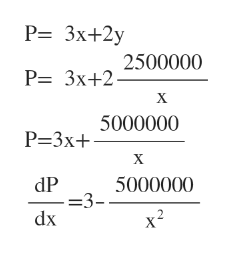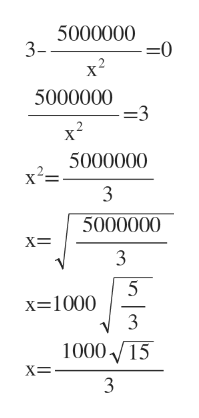# A rancher wants to fence in an area of 2500000 square feet in a rectangular field and then divide it in half with a fence down the middle, parallel to one side.What is the shortest length of fence that the rancher can use?

Question
269 views

A rancher wants to fence in an area of 2500000 square feet in a rectangular field and then divide it in half with a fence down the middle, parallel to one side.

What is the shortest length of fence that the rancher can use?

check_circle

Step 1

Let length of the sides are x and y. And the middle fence is parallel to x side.

So total fence needed = 3x+2y

Step 2

Given area = 2500000 square feet

So, xy= 2500000

y=2500000 / x

Plug this in the total perimeter. Then find dP/dxhelp_outlineImage TranscriptioncloseP= 3x+2y 2500000 P= 3x+2 X 5000000 P-3x+ X dP =3- dx 5000000 x2 fullscreen
Step 3

For minimum perimeter dP/dx=0 , from there w...help_outlineImage Transcriptionclose5000000 3- =0 x2 5000000 =3 x2 5000000 x2= 3 5000000 5 x=1000 3 1000 15 fullscreen

### Want to see the full answer?

See Solution

#### Want to see this answer and more?

Solutions are written by subject experts who are available 24/7. Questions are typically answered within 1 hour.*

See Solution
*Response times may vary by subject and question.
Tagged in
MathCalculus

### Other